# What calculations can I perform with Fractions Pro?

Fractions Pro performs calculations with fractions, mixed numbers, decimal and whole numbers.

The calculator performs the four basic arithmetic operations, calculates exponents, including negative exponents, and performs calculations with parentheses, including nested parentheses.

Here you can find some calculation examples.

### Example 1.

Calculate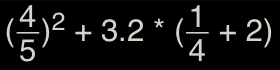How to input: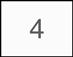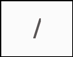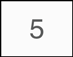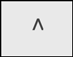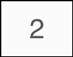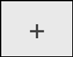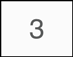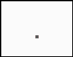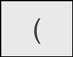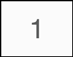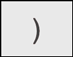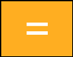#### Display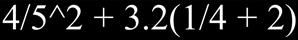### Example 2.

Calculate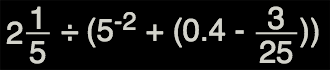How to input: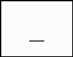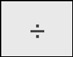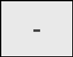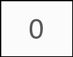#### Display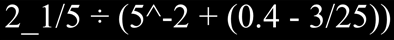Monday, December 4, 2023

Contact